## WitCH 3

First, a quick note about these WitCHes. Any reasonable mathematician looking at such text extracts would immediately see the mathematical flaw(s) and would wonder how such half-baked nonsense could be published. We are aware, however, that for teachers and students, or at least Australian teachers and students, it is not nearly so easy. Since school mathematics is completely immersed in semi-sense, it is difficult to know the rules of the game. It is also perhaps difficult to know how a tentative suggestion might be received on a snarky blog such as this. We’ll just say, though we have little time for don’t-know-as-much-as-they-think textbook writers, we’re very patient with teachers and students who are honestly trying to figure out what’s what.

Now onto WitCH 3, which follows on from WitCH 2, coming from the same chapter of Cambridge’s Specialist Mathematics VCE Units 3 & 4 (2018).* The extract is below, and please post your thoughts in the comments. Also a reminder, WitCH 1 and WitCH 2 are still there, awaiting proper resolution. Enjoy.

* Cambridge is a good target, since they are the most respected of standard Australian school texts. We will, however, be whacking other publishers, and we’re always open to suggestion. Just email if you have a good WitCH candidate, or crap of any kind you wish to be attacked.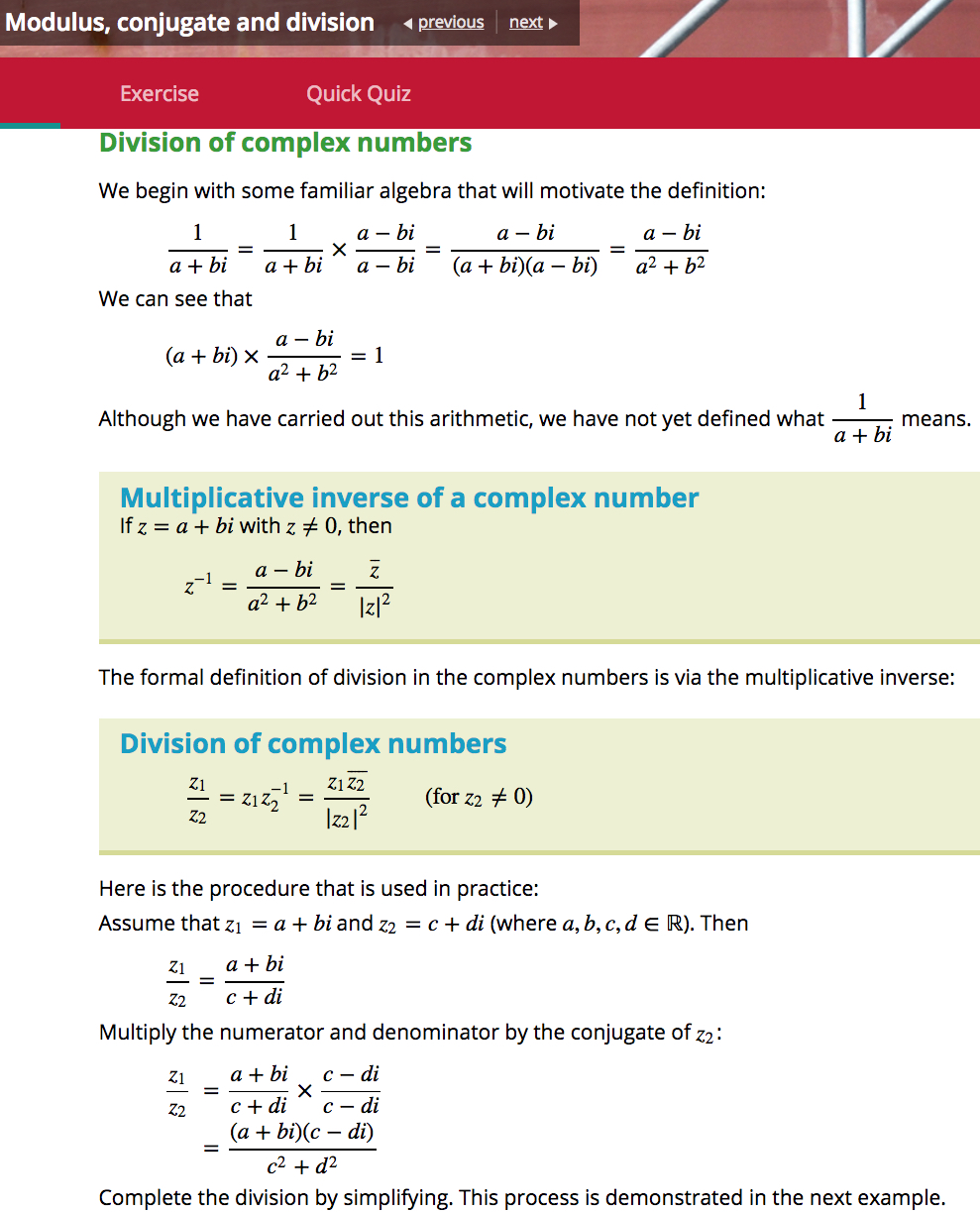## Update (06/02/19)

The above excerpt is indicative of the text’s entire chapter on complex numbers. It is such remarkably poor exposition, the foundations so understated and the direction so aimless, it is almost impossible to find one’s way back to sensible discussion.

Here is a natural framework for a Year 12 topic on complex numbers:

• First, one introduces a new numberfor which.
• One then defines complex numbers, and introduces the fundamental operations of addition and multiplication.
• One then at least states, and hopefully proves, the familiar algebraic properties for complex numbers, i.e. the field laws,and so forth. All these properties are obvious or straight-forward to prove, except for the existence of multiplicative inverses; one has to prove that given any non-zero complex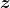there is another complex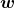with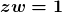.
• That is the basic complex algebra sorted, and then one can tidy up. This includes the definition of division, noting the essential role played by commutativity of multiplication.
• Then, comes the geometry of complex numbers, beginning with the definition and algebraic properties of the conjugate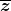and modulus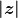,  the interpretation of these quantities in terms of the complex plane, and polar form.
• Finally, the algebra and geometry of complex numbers are related: the parallelogram interpretation of addition, the trigonometric-polar interpretation of multiplication, roots of complex numbers and so forth.

Must complex numbers be taught in this manner and in this order? No and yes. One obvious variation is to include a formal definition of a complex number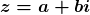as an ordered pair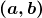; as Damo remarks below, this is done as an asterisked section in Fitzpatrick and Galbraith. Though unnervingly abstract, the formal definition has the non-trivial advantage of reinforcing, almost demanding, the interpretation of complex numbers as points in the complex plane. More generally, one can emphasise more or less of the theoretical underpinnings and, to an extent, change the ordering.

But, one can only change the ordering and discard the theory so much, and no more. Complex numbers are new algebraic objects, and defining and clarifying the algebra is critical, and this fundamentally precedes the geometry.

What is the Cambridge order? The text starts off well, introducingwith,  and then immediately goes off the rails by declaring that. Then, in brief, the text includes:

(a) an invalid treatment of the square roots of negative numbers;

(b) complex addition stated, presumably defined, with the inverse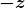introduced but not named;

(c) complex subtraction, followed by an almost invisible statement of the relevant field laws, none of which are proved or assigned as exercises;

(d) scalar multiplication;

(d) the complex plane and “the representation of the basic operations on complex numbers”;

(e) complex multiplication defined, with an almost invisible statement of field laws, none of which are proved or assigned as exercises, and with no mention of the question of multiplicative inverses;

(f) the geometry of multiplication by;

(g) the modulus of a complex number defined, with algebraic properties (including) stated and assigned as exercises;

(h) the conjugate of a complex number defined, with algebraic properties stated and either proved or assigned as exercises.

(h) Finally, as excerpted above, it’s on to reciprocals of complex numbers, multiplicative inverses in terms of modulus and conjugate, and division.

(i) This is followed by sections on polar form, de Moivre’s theorem and so forth;

(j) CAS garbage is, of course, interspersed throughout. (Which is not all Cambridge’s fault, but the text is no less ugly for that.)

At no stage in the text’s exposition is there any visible concern for emphasising or clarifying foundations, or for following a natural mathematical progression. There is too seldom an indication of what is being defined or assumed or proved.

What is the point? Yes, one can easily be overly theoretical on this topic, but this is Year 12 Specialist Mathematics. It is supposed to be special. The students have already been introduced to complex numbers in Year 11 Specialist. Indeed, much of the complex material in the Year 11 Cambridge text is repeated verbatim in the Year 12 text. Why bother? The students have already been exposed to the nuts and bolts, so why not approach the subject with some mathematical integrity, rather than just cutting and pasting aimless, half-baked nonsense?

Now, finally and briefly, some specific comments on the specific nonsense excerpted above.

• division of complex numbers has already appeared in the text, in the list of (unproved) properties of the modulus.
• the algebraic manipulation of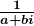is unfamiliar and unmotivated and, as is admitted way too late, is undefined. There is a place for such “let’s see” calculations – what mathematicians refer to as formal calculations –  but they have to be framed and be motivated much more carefully.
• There is no need here for a “let’s see” calculation. The critical and simple observation is thatis real. It is then a short step to realise and to prove that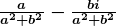acts as, and thus is, the multiplicative inverse of.
• Having finally admitted thathas not been defined, the text goes on to not define it again. The text states the multiplicative inverse of, but it is not clear whether this statement amounts to a definition or a conclusion.
• Division of complex numbers is then defined with needless subscripts and, more importantly, with no mention of the fundamental role of commutativity of multiplication.
• Throughout, the use of conjugate and modulus is muddying rather than clarifying.
• At no stage is it made clear whymakes sense in contrast to, for example, the non-sense of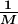for a matrix.